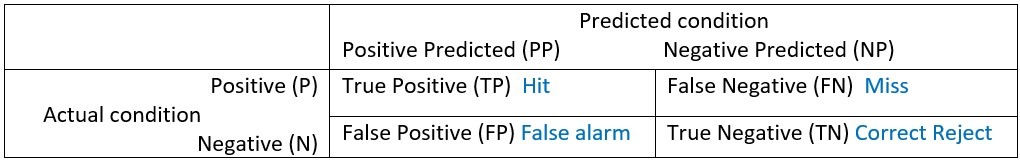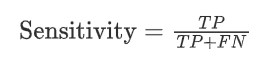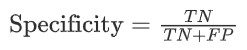×

Search anything:

# Confusion matrix and how accuracy is not the key always

#### Machine Learning (ML) Deep LearningConfusion matrix is a term in the field of machine learning which is generally associated with a lot of confusion on what it means, which is exactly what will be removed in this article as we understand confusion matrix.

1. Understanding Confusion Matrix
2. How accuracy is not the key always
3. Metrics Beyond Accuracy: Sensitivity & Specificity
4. Conclusion

# Understanding Confusion Matrix:

An evaluation of a classification algorithm's performance is done using a table called a confusion matrix. A confusion matrix depicts and summarises a classification algorithm's performance.Imagine we are solving a classification problem of a dog vs cat.
There are four fundamental components of the confusion matrix.

1. TP (True Positive): TP represents the number of cats who have been properly classified to be a cat, meaning the input is actually a cat.

2. TN (True Negative): TN represents the correct rejections, meaning the input is a dog.

3. FP (False Positive): FP represents the number of misclassified dogs but actually are cats, meaning a false alarm. FP is also known as a Type I error.

4. FN (False Negative): FN represents the number of dogs misclassified as cats but actually they are dogs. FN is also known as a Type II error.

All the above terms are utilised to specify the measurement parameters for the classifier.

The most commonly utilised performance metrics for classification according to these values are accuracy (ACC), precision (P), recall (R), and F1-score values.

Accuracy- The ratio of correctly classified cats (TP+TN) to the total number of dogs and cats, thus the formula to represent accuracy= TP+TN+FP+FN.

Precision- The ratio of correctly classified cats (TP) to the total number of cats (TP+FP).

Recall- The ratio of correctly classified cats (TP) divided by total number of cats and misclassified dogs. Recall is also called as sensitivity.

The F1 score, F score, or F measure. It is the harmoic mean of precision and sensitivity, it's a score which gives importance to both of these factors. The F1 score states the equilibrium between the precision and the recall.

# How accuracy is not the key always

Let's assume that the telecom firm's most critical problem is increasing 'churn', and the corporation is desperately trying to keep clients. The marketing manager makes the decision to roll out discounts and offers to all customers who are likely to leave in order to achieve this goal. Ideally, not a single 'churn' consumer should go unnoticed. Therefore, it is crucial that the model properly identify nearly all of the clients who 'churn'. It is acceptable if it predicts some of the 'non-churn' clients wrongly as 'churn', as the worst that may happen is that the business will give discounts to the customers who would have stayed otherwise.

Positive predicted Negative predicted
Positive 923 127
Negative 0 1050

Overall accuracy 93.952%

There are 1000 entries with the company currently. But only 540 of these 1000 are expected to "churn," according to the present model. Thus, only 540 out of 1000 clients, or only roughly 54%, will be projected by the model as "churning." This is particularly dangerous because the business won't be able to roll out offers to the remaining 46% of "churn" consumers and they might decide to do business with a rival!

# Metrics Beyond Accuracy: Sensitivity & Specificity:

So although the accuracy will be high in this case, the model only predicts 54% of churn cases correctly. In essence, what's happening here is that you care more about one class (class='churn') than the other. This is a very common situation in classification problems - you almost always care more about one class than the other. On the other hand, the accuracy tells you the model's performance on both classes combined - which is fine, but not the most important metric.
Hence, it is very crucial that you consider the overall business problem you are trying to solve to decide the metric you want to maximize or minimise.

This leads us to the two criteria that are most typically utilized to analyze a classification model- Sensitivity and Specificity ( Recall & Precision ).Using these two metrics we can analyze the classes of a classification problem with much more needed clarity. Thus in this case, the F1 score which states the equilibrium between the two is the a better parameter to judge against.

# Conclusion

In this article at OpenGenus, we understood the value of confusion matrix and its fundamental components. Then we learned about how accuracy cannot be used in every case. Lastly we talked about the metrics beyond accuracy.